# An algorithm for polylines outline construction

University of Maribor
Faculty of Electrical and Computer Sciences
Laboratory for Computer Graphics and Artificial Intelligence, Centre for Geometric Modelling
Smetanova 17, SI-2000 Maribor, Slovenia

Contents:
Abstract

Postscript version (`gzip'ed)

Abstract

The paper considers the problem of polylines outline construction. The algorithm works in three steps. At first, so called basic geometric buffers (BGB) are determined. Then, the intersection points between them are calculated. The intersection points are checked for containment in existed BGB. Only those intersection points not covered by any other BGB are used in the third part of the algorithm when the walk-about through the intersection points determines the final polylines outline.

1. Introduction

The problem of generation of an outline can be found in different applications. O'Rourke studied it as the key to safe robot arm movement [O'ROU93]. However, this problem is also of great importance in GIS applications. Let us consider a high-way (by the way, the high-way is in general represented by two parallel polylines) and let us suppose, we are interested in the area of propagation depending the terrain configuration. This information serves then to the road planner to decide where the noise barriers are needed.

The solution to this problem is quite simple at the first sight. Let us suppose we have a disk with a variable radius and we are moving the disk's centre point along the input polyline. The points of the moving disk arm the outline of the polyline. Theoretically the solution is obtained simply by the Minkowski sum (see [O'ROU93]), but practical implementation is not so simple. In this paper, the first prototype algorithmic solution to this problems is given.

2. The algorithm

The program input is a set of polylines (Figure 1a). Each polyline consist of several line segments, and two distances (di,1 is on the left side and d i,2 on the right side) are associated with each line segment li (see Figure 1a). The algorithm then calculates the outline as shown in Figure 1b.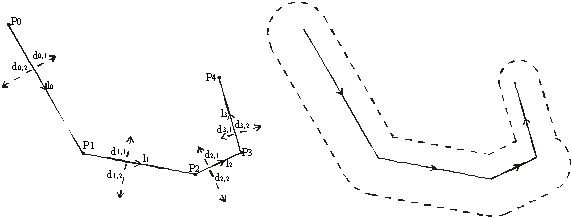Figure 1a) Input                                               Figure 1b) Output

The algorithm works in three steps:

1. The input polylines are separated into line segments and the outline (''we call it the basic geometric buffer  BGB'') is calculated for each of them.
2. The intersections between BGBs are determined.
3. The resulting outline is generated by performing a walk-about through the BGBs and the intersection points.
Each of these steps is divided into additional steps that will be considered in the continuation.

2.1. Determination of the basic geometric buffer

Each BGB has the same structure: it consists of two parallel line segments (li,1, l i,2) on the left and right side of the input line segment li and two semicircles (ci,1, ci,2) which connect these two line segments (see Figure 2).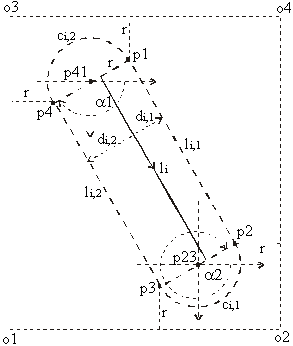Figure 2 BGB

The BGB is determined in three steps:

• determination of the left and right line segments (li,1, l i,2),
• calculating the data for both semicircles,
• setting the bounding rectangle (o1, o2, o3, o4) around the BGB.

2.1.1. Determination of the left and right line segments

This problem can be solved in two ways:

1. The first solution uses geometric transformations (translation and rotation) and it is easy to implement by the following steps:

• moving the line segment li shown in Figure 3a in the center of the co-ordinate system
• the line segment is rotated onto positive x axis for angle a (Figure 3b and Equation 1)
• the point P''i+1 is calculated (see Equation 2)
• it is easy now to calculate all information needed to set up the BGB (see Figure 3c)
• at the end, the BGB is returned to its original position.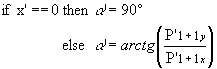Equation 1a Because function arctg returns only angles for positive x we added condition (see Equitation 1b):Equation 1b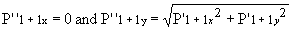Equation 2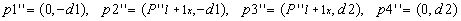Equation 3Equation 4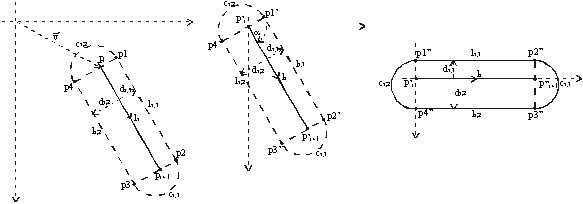Figure 3a) Original position               Figure 3b) Moved position             Figure 3c) Rotated position

The following operations are needed for obtaining this solution:

2. The second possibility calculates all data directly on the line segment. Its implementation is more complicated but also more efficient:
• The deviations (xr1, yr1, xr2, yr2) from the line segment li are calculated at first. This task is performed in two different ways according the position of points Piy, Pi+1: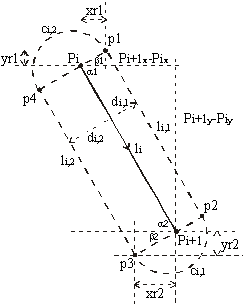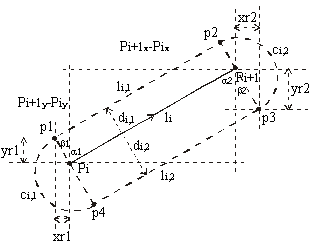Figure 4 Piy < Pi+1y                              Figure 5 Piy > Pi+1y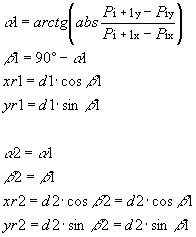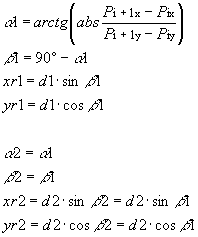Equation 5                                                  Equation 6

Then these deviations are added to the line segment to get the left and right line segments (li,1, li,2). There are four different possibilities but only two of them are needed in each case:
1. Piy < Pi+1y (see Equation 7a)
2. Piy > Pi+1y (see Equation 7b)
3. Pix < Pi+1x (see Equation 7c)
4. Pix > Pi+1x (see Equation 7d)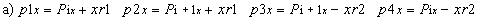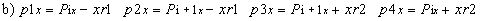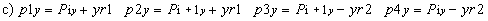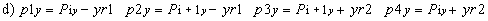Equation 7
We need only:

2.1.2. Determination of semicircles

To determine the semicircle data, the radius, two center points and two initial angles are needed (see Figure 2). Radius and center points are easy to be determined (see Equation 8 and Equation 9).

Radius: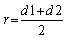Equation 8

Central points: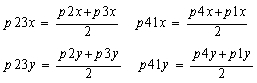Equation 9

A bit more care should be given to determining of the initial angles a1 and a2. In fact, it is enough to calculate only one angle. The other one is obtained by adding 180° to the calculated angle.

By the help of the line segment connecting the beginning and the end of the semicircle (see Figure 2) the initial angle is calculated(see Figure 6 and Equation 10):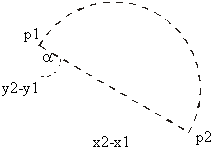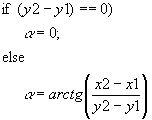Figure 6 How to get angle a                                 Equation 10

There exist two possibilities to calculate initial angles a1 and a2 (see Figure 2):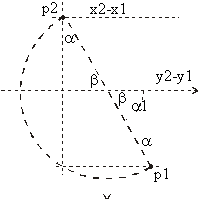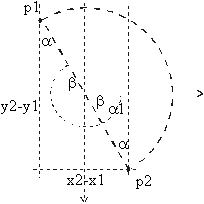Figure 7 (y2 > y1) or (y2 = y1 and x2 > x1)     Figure 8 (y2 < y1) or (y2 = y1 and x2 < x1)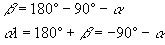Equation 11                                               Equation 12

2.1.3. Determination of the banding rectangle

The algorithm does not determine the smallest banding rectangle because its determination would take a lot of time. In that case, we would need trigonometric operations, multiplications, square root operations, division, additions and subtractions. So we use a method where we needed only 2 additions and 2 subtractions (see Figure 2), but the banding rectangle is a bit larger than necessary.

At first, the minimum and maximum value of co-ordinates p1, p2, p3, p4 in each co-ordinate direction are determined. (minX, minY, maxX, maxY)

Then the banding rectangle is stretched for the value of semicircle radius in each direction (see Figure 2 and Equation 13)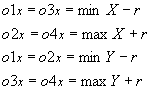Equation 13

2.2. Finding the intersection points between BGB

To reduce the number of calculations, the banding rectangles are used. If two banding rectangles overlap, it is possible that intersection points exist and the future actions are necessary.

In Figure 9, we can see all possible intersections that can occur:

• two line segments (see Figure 9, point P1),
• one line segment and one semicircle (see Figure 9, point P2),
• two semicircles (see Figure 9, point P3).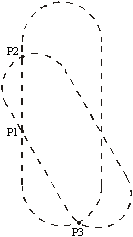Figure 9 Possible intersection points

Let us consider these cases briefly.

2.2.1. Intersection of two line segment

The input data consists of beginning and ending points of two line segments (line segment1 [(x11,y11), (x21,y21)]; line segment2 [(x12,y12), (x22,y22)]).
This case is simple enough that it does not need additional observation. For the purpose of completeness let us give just the final formula (see Equation 14)Equation 14
After using Equatoin 14, the obtained intersection point has to be checked if it lies on both line segments, because here we only find the intersection point between two lines and it is possible that it do not lie on line segments as we need.

2.2.2. An intersection of line segment and a semicircle

The input data consists of beginning and ending points of a line segment [(x11, y11), (x21, y21)], and data describing a semicircle (center point (xs, ys), radius (r) and initial angle (a )). There are three possible solutions (see Figure 10):

• no intersection (a)
• one intersection point (b)
• two intersection points (c)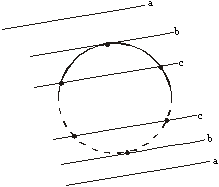Figure 10 Possible intersection points line-circle

Basic formulae are described in Equation 15.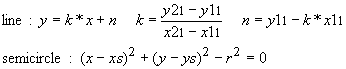Equation 15

If x21 = x11 then the Equation 16 is obtained.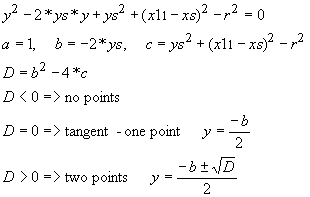Equation 16

In other case, the Equation 17 is applied.Equation 17

These equations actually consider the intersection between a line and a circle. Therefore, it has to be checked if the intersection points lay on the line segment and the semicircle indeed.

2.2.3. Intersections between semicircles

At first, the intersection points between two circles determined by center points [(xs1, xs1), (xs2, ys2)], radii (r1, r2) and initial angles (a 1, a 2) are determined.
The circles are expressed by formulae in Equation 18.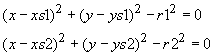Equation 18

First, the distance (d) between center points and absolute difference (drad) between radii are calculated (see Equation 19).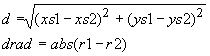Equation 19

With these two parameters, the following cases should be considered:

• If (d = 0) and (drad = 0) then the circles cover partially each other and we get the point in the middle of their covering (see Figure 11 and Equation 20).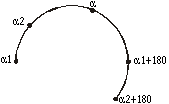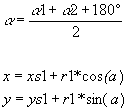Figure 11 How to get middle point                          Equation 20
• If (d < drad) (see Figure 12a) or (d > r1+r2) (see Figure 12b) then the circles do not have any common points.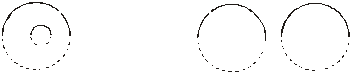Figure 12a One circle is inside the other      Figure 12b Circles have no common surface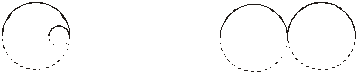Figure 13a One circle is inside the other              Figure 13b Circles have no common surface

• If the center points of the circles lie on the same vertical line (xs1 = xs2), the intersection point is obtained by the Equation 21:

•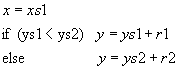Equation 21

• If they do not lie on the same vertical line then Equation 22 is applied (see Figure 14):

•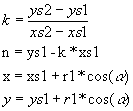Figure 14 They have one common point                              Equation 22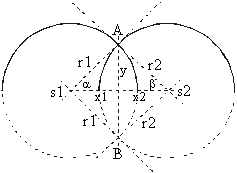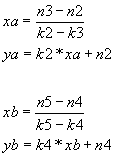Figure 15 They have two common points                          Equation 23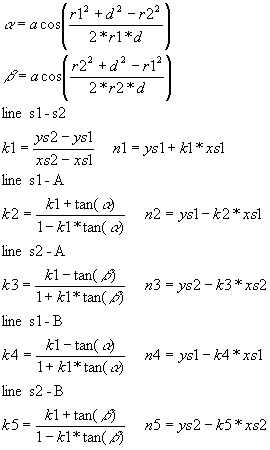Equation 24

At the end, it has to be checked if the intersection point (or points) lies on both semicircles.

2.2.4. Elimination of covered intersection points

After obtaining the intersection points, we have to remove those which are inside any BGB. Let us see the example in Figure 16.

The final outline goes through intersection points q1, q2, q3, q6, q7, q1.
Points q5 and q4, which are covered with BGB are not a part of the solution, so we delete them (see Figure 16).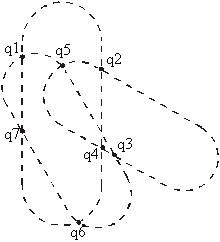Figure 16 Found intersection points

This problem is naturally separated into two smaller problems:

• the point is inside of a semicircle
• the point is inside of a rectangle (p1, p2, p3, p4)

2.3. Construction of the common outline

When all necessary data are obtained the common outline is obtained. It consists of exactly one outer border (named loop) and zero or finite number of inner borders (named rings) [MORT85]. The rings represent the holes in the outline.

If there exist non-covered intersection points, we continue with the following:

1. The algorithm takes the intersection point with the smallest x co-ordinate. If there are more such points, the one with the largest y co-ordinate is accepted and remembered. This point for sure belongs to the loop.
2. The direction of movement is determined.
3. The walk-about through the intersection points is performed until the remembered point is met. Visited intersection points are marked as used. If non-used intersection points still exist, the algorithm returns to step 1, but this time, the ring is going to be constructed.

2.3.1. Determine the direction of movement

In our case, the clock-wise direction of movement is chosen. At the first point the right direction has to be calculated.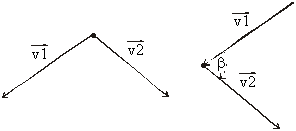Figure 17 Direction of next movement
The direction is determined by the vector product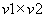(see Figure 17).

Because we travel in the clockwise direction, we always follow the vector which lies in the left halfplane of the other vector (see Figure 17). With other words, if the vector product is positive the first vector (v1) is followed and if it is negative the direction of the second vector (v2) is applied.

The walk-about is relatively easy to implement:

• We look if there are any intersection points on the curve on which we are now.
1. If there is no intersection point we take whole curve to the ending point and start with the next curve (see Figure 18).
2. If there are intersection points we find the nearest one and take the curve to this point and then move in this point. Then we see if we are back in the first intersection point.
1. 2.1. If we came back we finished with this loop and start the with next one (back to point 1 in section 2.3) (see Figure 18).
2.2. If we did not come back then we start with the next curve. We continue travelling the curve that intersects the curve we have just travelled now and we continue with this algorithm (see Figure 18).
Let us see this on example (see Figure 18):Figure 18 Walk-about

The walk-about starts at point q1. By the help of the vector product, the direction of vector v is chosen. The next intersection point is q2. Here we jump on the second BGB and follow its outline until intersection point q3 is found. Here again the BGB is changed. The process continues until q1 is meet again.

• If no intersection points are found, two cases exist:
1. All BGBs are outside each other (see Figure 19) and the result consists of these individual BGBs.
2. There is the possibility that some BGBs are ''eaten'' by the others. The containment test has to be applied to remove the ''eaten'' BGBs (see Figure 20).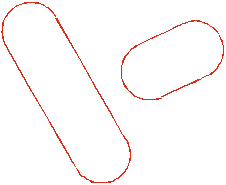Figure 19 BGBs are outside each other                Figure 20 BGB is eaten by other BGB
Figure 21, Figure 22 and Figure 23 show the input, the intermediate and the final state of the working example.Figure 21 Input lines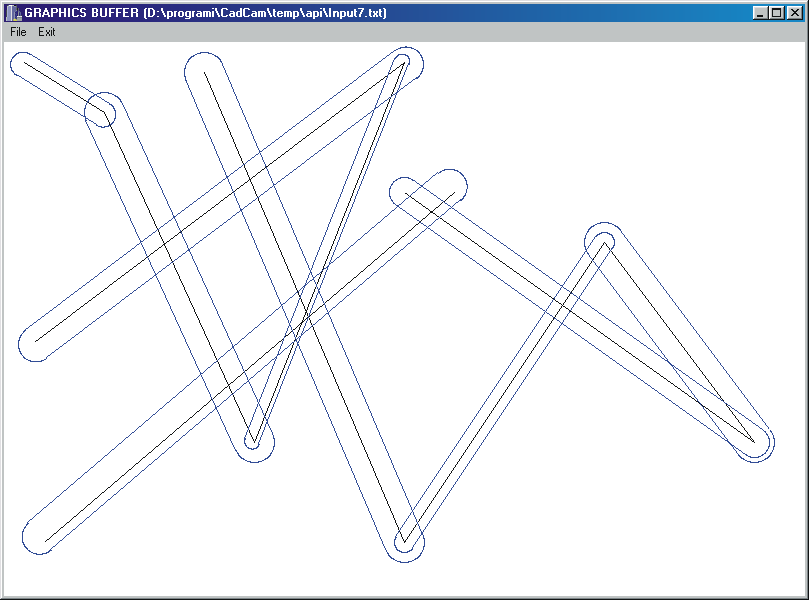Figure 22 Intermediate state - BGBs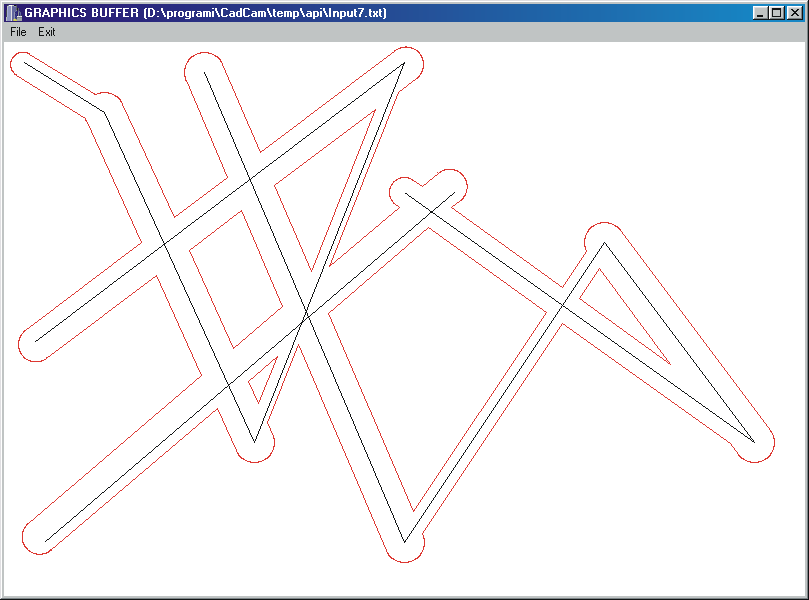Figure 23 Final state  loop and rings

3. Conclusion

The paper considers an outline construction algorithm. The most difficult task of the algorithm considering spent CPU time is the elimination of found intersection points covered by BGBs. At this moment, we do not use any acceleration techniques (plane subdivision). However, the described algorithm is being used successfully at the Faculty of Civil Engineering for the road design. The implementation turns out as being stable and quick enough for their purposes. The algorithm has been implemented in C++ and can be found at http://www.uni-mb.si.

Literature:

[MORT85] Mortenson, M.E., ''Geometric Modelling'', John Wiley & Sohns, 1985.

[O'ROU93] O'Rourke, J., ''Computational Geometry in C'', Cambridge University Press, 1993